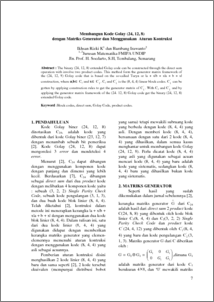# MEMBANGUN KODE GOLAY (24, 12, 8) DENGAN MATRIKS GENERATOR DAN MENGGUNAKAN ATURAN KONTRUKSI

RIZKI, IKHSAN and irawanto, bambang (2010) MEMBANGUN KODE GOLAY (24, 12, 8) DENGAN MATRIKS GENERATOR DAN MENGGUNAKAN ATURAN KONTRUKSI. Jurnal Matematika, 13 (1). pp. 7-14.Preview
PDF
77Kb

## Abstract

The binary (24, 12, 8) extended Golay code can be constructed through the direct sum operation with involve two product codes. This method form the generator matrix framework of the (24, 12, 8) Golay code that is based on the so-called Turyn or |a + x|b + x|a + b + x| construction, where a,b C1 and x C . C1 and C is the (8, 4, 4) linear block codes. C can be gotten by applying construction rules to get the generator matrix of C . With C1 and C and by applying the generator matrix framework of the (24. 12, 8) Golay code get the binary (24, 12, 8) extended Golay code.

Item Type: Article Q Science > QA Mathematics Faculty of Science and Mathematics > Department of Mathematics 26867 INVALID USER 19 Apr 2011 15:42 19 Apr 2011 15:42

Repository Staff Only: item control page